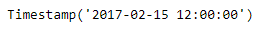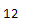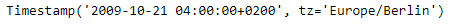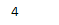# Python | Pandas Timestamp.hour

• Last Updated : 08 Jan, 2019

Python is a great language for doing data analysis, primarily because of the fantastic ecosystem of data-centric python packages. Pandas is one of those packages and makes importing and analyzing data much easier.

Pandas` Timestamp.hour` attribute return the value of the hour for the given Timestamp object.

Syntax : Timestamp.hour

Parameters : None

Return : hour

Example #1: Use `Timestamp.hour` attribute to find out the hour value in the given Timestamp object.

 `# importing pandas as pd``import` `pandas as pd`` ` `# Create the Timestamp object``ts ``=` `pd.Timestamp(``2017``, ``2``, ``15``, ``12``)`` ` `# Print the Timestamp object``print``(ts)`

Output :Now we will use the `Timestamp.hour` attribute to find out hour value in the given Timestamp object.

 `# return the hour value``ts.hour`

Output :As we can see in the output, the `Timestamp.hour` attribute has returned 12 as the hour value for the given Timestamp object.

Example #2: Use `Timestamp.hour` attribute to find out the hour value in the given Timestamp object.

 `# importing pandas as pd``import` `pandas as pd`` ` `# Create the Timestamp object``ts ``=` `pd.Timestamp(year ``=` `2009``, month ``=` `10``, day ``=` `21``,``                     ``hour ``=` `4``, tz ``=` `'Europe/Berlin'``)`` ` `# Print the Timestamp object``print``(ts)`

Output :Now we will use the `Timestamp.hour` attribute to find out hour value in the given Timestamp object.

 `# return the hour value``ts.hour`

Output :As we can see in the output, the `Timestamp.hour` attribute has returned 4 as the hour value for the given Timestamp object.

My Personal Notes arrow_drop_up